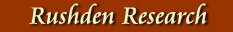WEA Study Notes & Survey by P. Robinson, 1982
Inns, Hotels & Public Houses
1847 -1940 - from Trade Directories

 1847 1854 1861 1870 1877 1885 1890 1894 1903 1910 1920 1931 1936 1940 Coach & Horses X X X X X Compass Inn X The Feathers X X X X New Inn (later Railway Hotel) X X X Oakley Arms X X X X X X X X X Queen Victoria Hotel X X X X X X Railway Hotel (see New Inn) X X X X X X Rose & Crown X X X X X X X X X X X X Rushden Hotel, Coffee Tavern & Public Hall X X X X X X Unicorn X X X X X Waggon & Horses X X X X X X X X X X X X X X Waverley Temperance Hotel X X X X X Wheatsheaf X X X X X X X X X X X X X X

 Other tables showing the distribution of trades based on entries in various Trade Directories